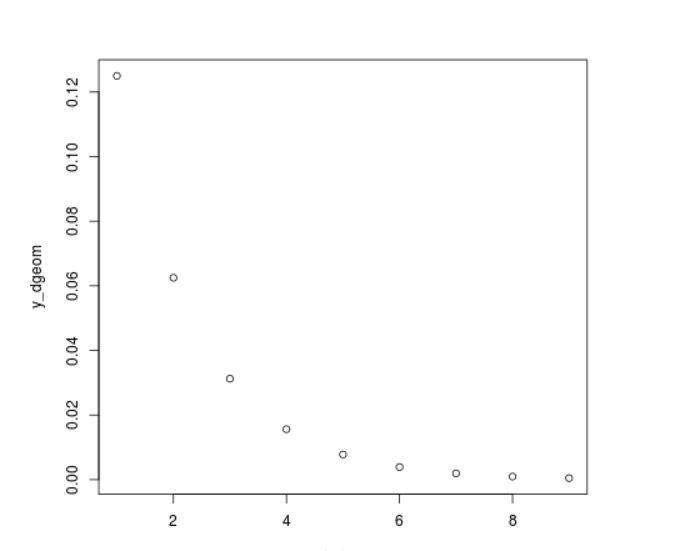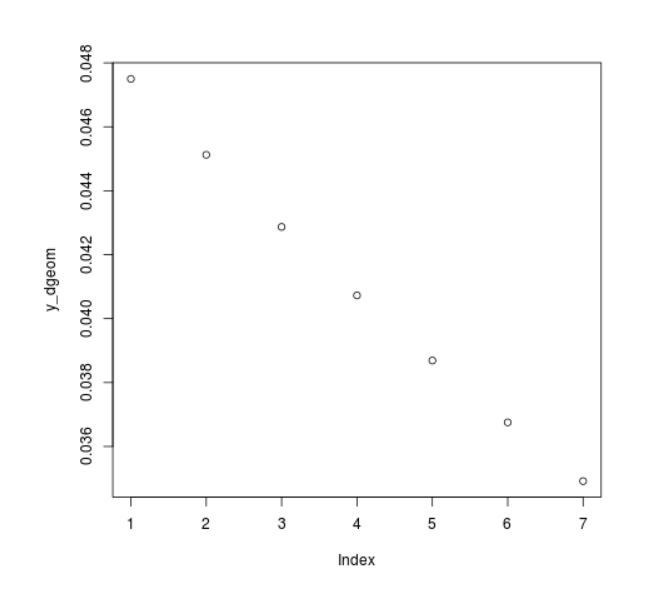# Plot a Geometric Distribution Graph in R Programming – dgeom() Function

`dgeom()` function in R Programming is used to plot a geometric distribution graph.

Syntax: dgeom(x, prob)

Parameters:

• prob: prob of the geometric distribution
• x: x values of the plot

Example 1:

 `# R program to illustrat ` `# dgeom function to plot ` ` `  `# Specify x-values for dgeom function ` `x_dgeom <``-` `seq(``2``, ``10``, by ``=` `1``)     ` ` `  `# Apply dgeom function  ` `y_dgeom <``-` `dgeom(x_dgeom, prob ``=` `0.5``)     ` ` `  `# Plot dgeom values  ` `plot(y_dgeom)                                                                          `

Output:Example 2:

 `# R program to illustrate ` `# dgeom() function to plot ` ` `  `# Specify x-values for dgeom function ` `x_dgeom <``-` `seq(``1``, ``7``, by ``=` `1``)     ` ` `  `# Apply dgeom function  ` `y_dgeom <``-` `dgeom(x_dgeom, prob ``=` `0.05``)     ` ` `  `# Plot dgeom values  ` `plot(y_dgeom)      `

Output:My Personal Notes arrow_drop_upCheck this out nerdboinet

If you like GeeksforGeeks and would like to contribute, you can also write an article using contribute.geeksforgeeks.org or mail your article to contribute@geeksforgeeks.org. See your article appearing on the GeeksforGeeks main page and help other Geeks.

Please Improve this article if you find anything incorrect by clicking on the "Improve Article" button below.

Article Tags :

Be the First to upvote.

Please write to us at contribute@geeksforgeeks.org to report any issue with the above content.